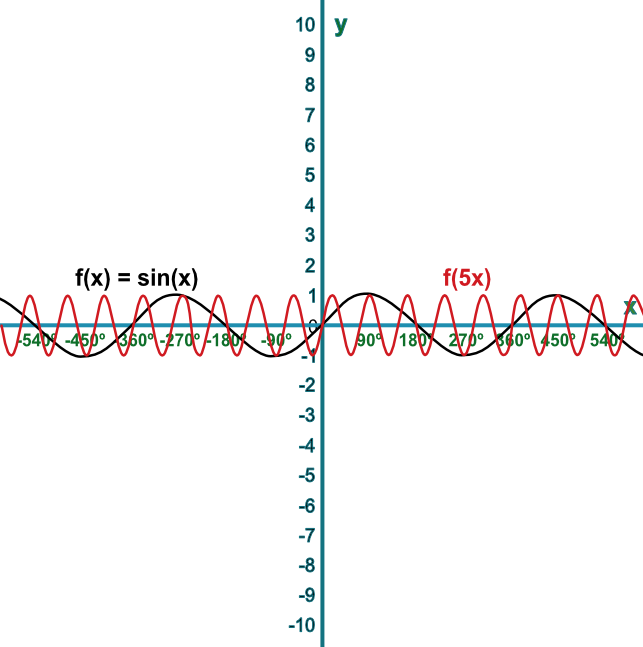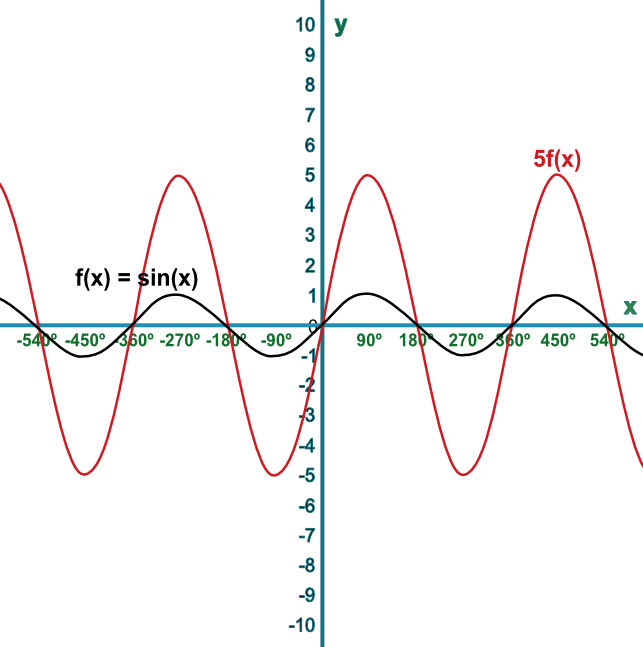Sketch Stretching of Functions

## Sketch Stretching of Functions

Graphs can be stretched horizontally and vertically by applying scale factors. If the factor is applied before the function is evaluated, then the stretching takes place parallel to the x-axis, but the amount of stretching is a reciprocal of the factor:

Mapping f(x) onto f(ax) stretches a graph by factor frac(1)(a) on the x-axis.

If the factor is applied after the function is evaluated, then the stretching takes place parallel to the y-axis:

Mapping f(x) onto af(x) stretches a graph by factor a on the y-axis.

## Example 1

Sketch the graph of the function f(x)=sin(x). On the same graph, sketch f(5x).

Describe the transformation.

A multiple applied before the function is evaluated will expand by a scale factor of the reciprocal of the multiple along the x-axis.

Answer: The curve is enlarged parallel to the x-axis with a scale factor of frac(1)(5)## Example 2

Sketch the graph of the function f(x)=sin(x). On the same graph, sketch 5f(x).

Describe the transformation.

A multiple applied after the function is evaluated will expand by a scale factor of the multiple in the direction of the y-axis.

Answer: The curve is enlarged parallel to the y-axis with a scale factor of 5